## Notations

The alphabetical order notations below if for your reference in this tutorial. You can refer back later if necessary.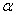= Portion of distance per trip (half of the cycle) that normally traveled by all passengers (percent)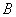= Total benefit after conversion into present time value (\$)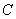= Total cost after conversion into present time value (\$)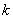= Interest rate (percent)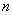= Period of usage year that a bus can be operated from purchasing time until is sold again (years)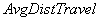= average distance travel of by all passengers (km)

One cycle is from origin station to destination station and back to origin station again.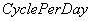= Number of cycles (origin->destination->origin) that a bus can operate in one day (cycle/day)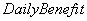= Benefit of a bus system from the operation per day (\$/day)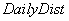= Total distance that a bus will operate in one day (km/day)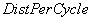= Total distance that a bus will operate in one cycle (km/cycle).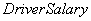= Monthly salary of a bus driver (\$/month)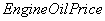= Price of engine oil per can (\$/can)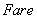= Payment that passenger that received by the system (\$/passenger)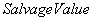= Price of the bus after usage year (\$)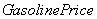= Gasoline price per liter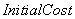= Purchasing cost of one bus (\$)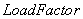= Average fluctuation of demand in that particular route (1/cycle)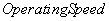= Average speed of operation that include the effect of traffic congestion (km/hour)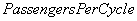= Number of passengers in one cycle (passengers/cycle).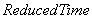= Time reduction due stopping time to boarding and alighting of passengers along the way and some reduce time due to slowing down to get the passengers (hour/passenger).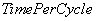= Total number of hours spends by a bus to operate in one cycle.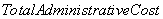= Total yearly administrative (tax, vehicle inspection, administrators) and simple maintenance such as cleaning and painting (\$/year)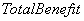= Total benefit in one year (\$/year)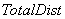= Total distance of bus operation throughout one year (km/year)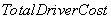= Bus driver salary for one year (\$/year)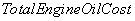= Total cost due to engine oil for the operation of the bus (\$/year)= Total cost due to gasoline for the operation of the bus (\$/year)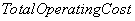= Total operating cost in one year (\$/year) which include all operation, administrative and maintenance cost but exclude initial purchasing cost.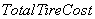= Total cost due to tire for the operation of the bus (\$/year)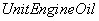= Total distance of that a bus can run using one can of engine oil (km/can)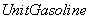= Total distance of that a bus can run using one liter of gasoline (km/liter)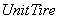= Total distance of that one tire in a bus can run until need to be replaced (km)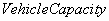= Total number of passenger can ride a bus (passengers)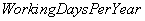= Number of working day per year that the bus can be operated

Preferable reference for this tutorial is

Teknomo, Kardi (2006) Tutorial on Feasibility Study. http://people.revoledu.com/kardi/tutorial/What-If-Analysis/index.html